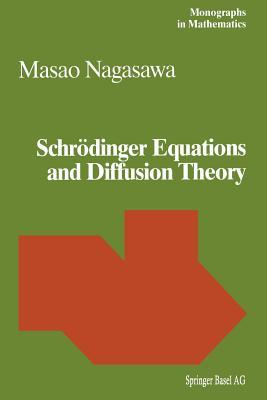# Schrodinger Equations and Diffusion TheoryAuthor: M Nagasawa

Format: Paperback

Pages: 323

ISBN: 9783034896849

Publisher: Birkhauser

Release Date: September 27, 2012

Description du Livre

Schrodinger Equations and Diffusion Theory addresses the question "What is the Schrodinger equation?" in terms of diffusion processes, and shows that the Schrodinger equation and diffusion equations in duality are equivalent. In turn, Schrodinger's conjecture of 1931 is solved. The theory of diffusion processes for the Schrodinger equation tell us that we must go further into the theory of systems of (infinitely) many interacting quantum (diffusion) particles.
The method of relative entropy and the theory of transformations enable us to construct severely singular diffusion processes which appear to be equivalent to Schrodinger equations.
The theory of large deviations and the propagation of chaos of interacting diffusion particles reveal the statistical mechanical nature of the Schrodinger equation, namely, quantum mechanics.
The text is practically self-contained and requires only an elementary knowledge of probability theory at the graduate level.
"

Commentaires
Alphonse Ratté
Je tiens à vous remercier personnellement pour vos livres exceptionnels. C’est vraiment merveilleux.